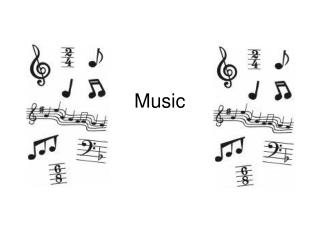DownloadDownload PresentationMusic

Music

Télécharger la présentationMusic

- - - - - - - - - - - - - - - - - - - - - - - - - - - E N D - - - - - - - - - - - - - - - - - - - - - - - - - - -
Presentation Transcript

1. Music

2. 1. Which note is a whole note? A. B. C. D.

3. 2. How many beats does a whole note get? A. B. C. D. 1 2 4 3

4. 3. Which note is a half note? A. B. C. D.

5. 4. How many beats does a half note get? A. B. C. D. 1 2 4 3

6. 5. Which note is a quarter note? A. B. C. D.

7. 6. How many beats does a quarter note get? A. B. C. D. 1 2 4 3

8. 7. Which note is a dotted half note? A. B. C. D.

9. 8. How many beats does a dotted-half note get? A. B. C. D. 1 2 4 3

10. 9. Which note is an eighth note? A. B. C. D.

11. 10. How many beats do two eighth notes get? A. B. C. D. 1 2 4 3

12. 11. Name the spaces A. B. EGBDF FACE C. D. GBDFA ACEG

13. 12. Name the lines A. B. EGBDF FACE C. D. GBDFA ACEG

14. 13. Name the spaces A. B. EGBDF FACE C. D. GBDFA ACEG

15. 14. Name the lines A. B. EGBDF FACE C. D. GBDFA ACEG